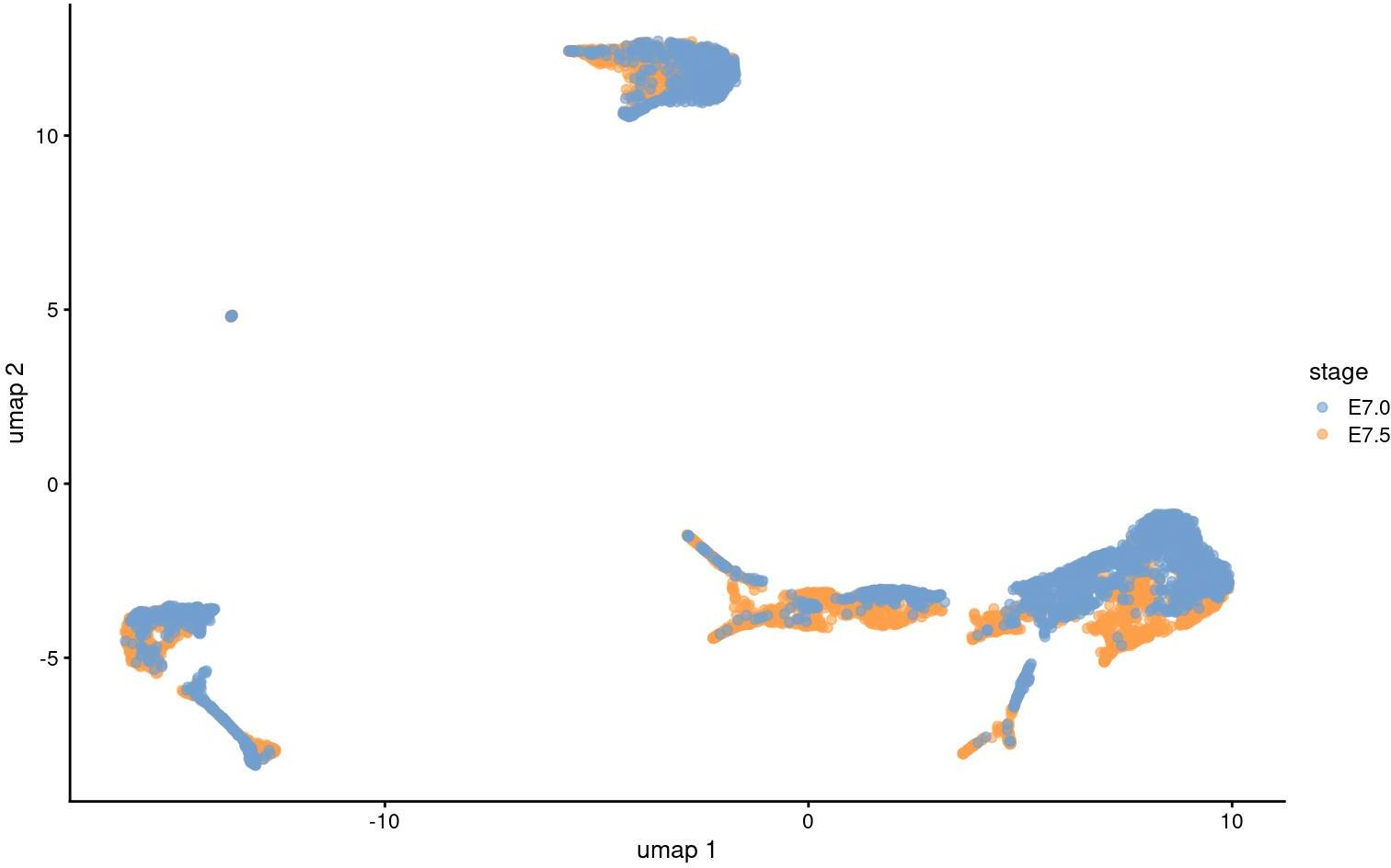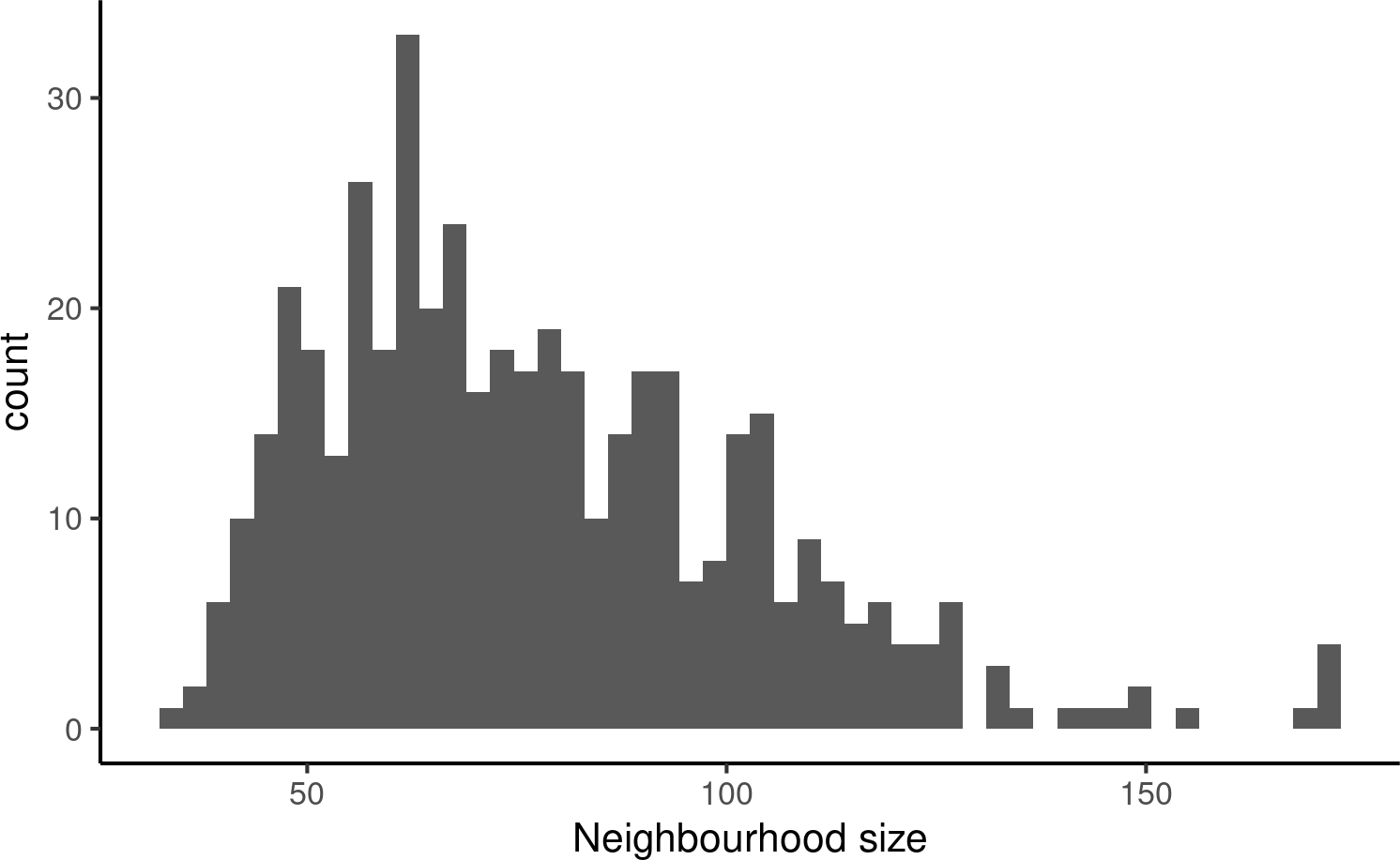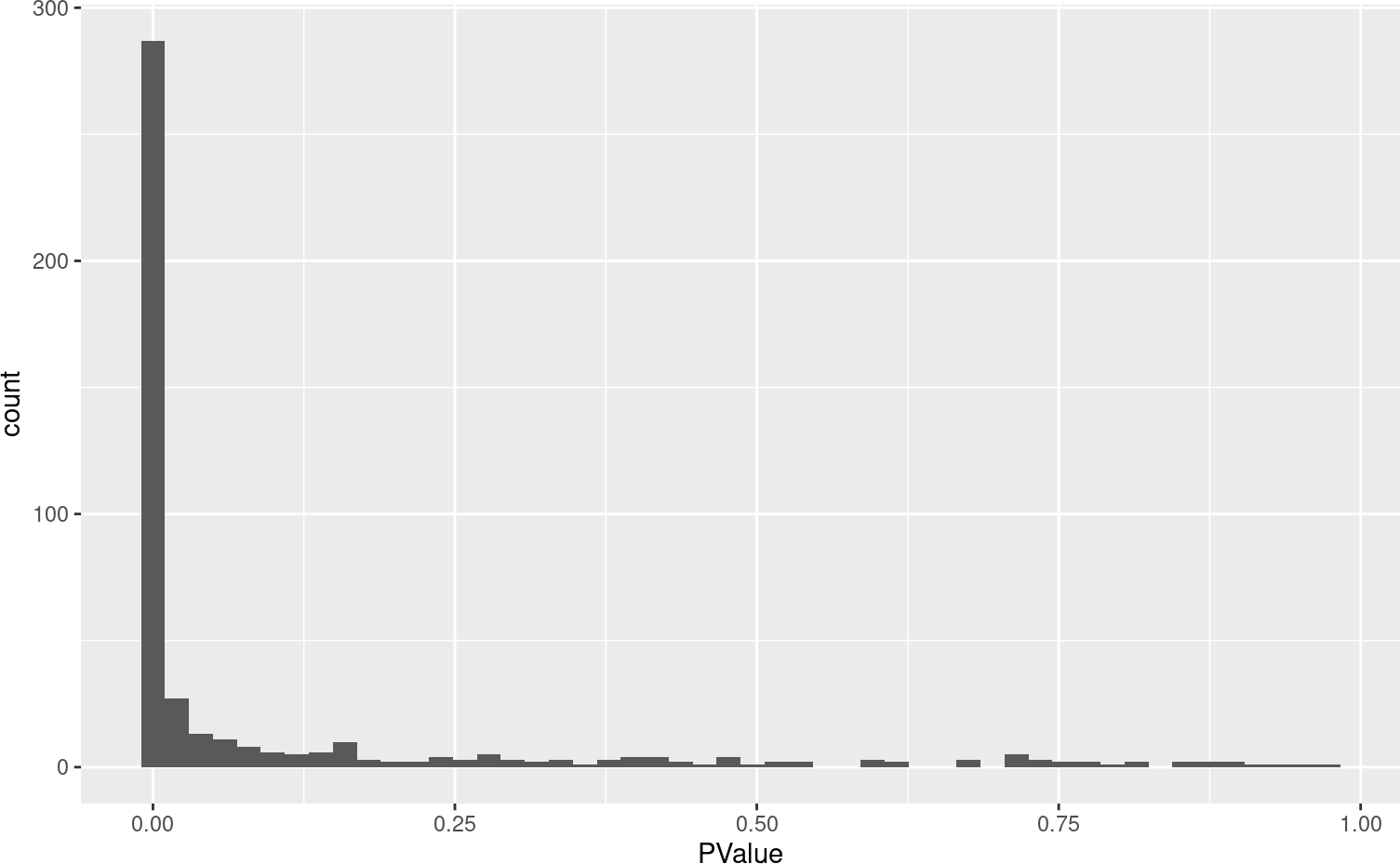library(miloR)
library(SingleCellExperiment)
library(scater)
library(scran)
library(dplyr)
library(patchwork)
library(MouseGastrulationData)

For this vignette we will use the mouse gastrulation single-cell data from Pijuan-Sala et al. 2019. The dataset can be downloaded as a SingleCellExperiment object from the MouseGastrulationData package on Bioconductor. To make computations faster, here we will download just a subset of samples, 4 samples at stage E7 and 4 samples at stage E7.5.

This dataset has already been pre-processed and contains a pca.corrected dimensionality reduction, which was built after batch correction using fastMNN.

select_samples <- c(2,  3,  6, 4, #15,
# 19,
10, 14#, 20 #30
#31, 32
)
embryo_data = EmbryoAtlasData(samples = select_samples)
embryo_data
## class: SingleCellExperiment
## dim: 29452 7558
## assays(1): counts
## rownames(29452): ENSMUSG00000051951 ENSMUSG00000089699 ...
##   ENSMUSG00000096730 ENSMUSG00000095742
## rowData names(2): ENSEMBL SYMBOL
## colnames(7558): cell_361 cell_362 ... cell_29013 cell_29014
## colData names(17): cell barcode ... colour sizeFactor
## reducedDimNames(2): pca.corrected umap
## mainExpName: NULL
## altExpNames(0):

# 2 Visualize the data

We recompute the UMAP embedding for this subset of cells to visualize the data.

embryo_data <- embryo_data[,apply(reducedDim(embryo_data, "pca.corrected"), 1, function(x) !all(is.na(x)))]
embryo_data <- runUMAP(embryo_data, dimred = "pca.corrected", name = 'umap')

plotReducedDim(embryo_data, colour_by="stage", dimred = "umap")We will test for significant differences in abundance of cells between these stages of development, and the associated gene signatures.

# 3 Differential abundance testing

## 3.1 Create a Milo object

For differential abundance analysis on graph neighbourhoods we first construct a Milo object. This extends the SingleCellExperiment class to store information about neighbourhoods on the KNN graph.

embryo_milo <- Milo(embryo_data)
embryo_milo
## class: Milo
## dim: 29452 6875
## assays(1): counts
## rownames(29452): ENSMUSG00000051951 ENSMUSG00000089699 ...
##   ENSMUSG00000096730 ENSMUSG00000095742
## rowData names(2): ENSEMBL SYMBOL
## colnames(6875): cell_361 cell_362 ... cell_29013 cell_29014
## colData names(17): cell barcode ... colour sizeFactor
## reducedDimNames(2): pca.corrected umap
## mainExpName: NULL
## altExpNames(0):
## nhoods dimensions(2): 1 1
## nhoodCounts dimensions(2): 1 1
## nhoodDistances dimension(1): 0
## graph names(0):
## nhoodIndex names(1): 0
## nhoodExpression dimension(2): 1 1
## nhoodReducedDim names(0):
## nhoodGraph names(0):
## nhoodAdjacency dimension(2): 1 1

## 3.2 Construct KNN graph

We need to add the KNN graph to the Milo object. This is stored in the graph slot, in igraph format. The miloR package includes functionality to build and store the graph from the PCA dimensions stored in the reducedDim slot. In this case, we specify that we want to build the graph from the MNN corrected PCA dimensions.

For graph building you need to define a few parameters:

• d: the number of reduced dimensions to use for KNN refinement. We recommend using the same $$d$$ used for KNN graph building, or to select PCs by inspecting the scree plot.
• k: this affects the power of DA testing, since we need to have enough cells from each sample represented in a neighbourhood to estimate the variance between replicates. On the other side, increasing $$k$$ too much might lead to over-smoothing. We suggest to start by using the same value for $$k$$ used for KNN graph building for clustering and UMAP visualization. We will later use some heuristics to evaluate whether the value of $$k$$ should be increased.
embryo_milo <- buildGraph(embryo_milo, k = 30, d = 30, reduced.dim = "pca.corrected")

Alternatively, one can add a precomputed KNN graph (for example constructed with Seurat or scanpy) to the graph slot using the adjacency matrix, through the helper function buildFromAdjacency.

## 3.3 Defining representative neighbourhoods on the KNN graph

We define the neighbourhood of a cell, the index, as the group of cells connected by an edge in the KNN graph to the index cell. For efficiency, we don’t test for DA in the neighbourhood of every cell, but we sample as indices a subset of representative cells, using a KNN sampling algorithm used by Gut et al. 2015.

As well as $$d$$ and $$k$$, for sampling we need to define a few additional parameters:

• prop: the proportion of cells to randomly sample to start with. We suggest using prop=0.1 for datasets of less than 30k cells. For bigger datasets using prop=0.05 should be sufficient (and makes computation faster).
• refined: indicates whether you want to use the sampling refinement algorithm, or just pick cells at random. The default and recommended way to go is to use refinement. The only situation in which you might consider using random instead, is if you have batch corrected your data with a graph based correction algorithm, such as BBKNN, but the results of DA testing will be suboptimal.
embryo_milo <- makeNhoods(embryo_milo, prop = 0.1, k = 30, d=30, refined = TRUE, reduced_dims = "pca.corrected")

Once we have defined neighbourhoods, we plot the distribution of neighbourhood sizes (i.e. how many cells form each neighbourhood) to evaluate whether the value of $$k$$ used for graph building was appropriate. We can check this out using the plotNhoodSizeHist function.

As a rule of thumb we want to have an average neighbourhood size over 5 x N_samples. If the mean is lower, or if the distribution is

plotNhoodSizeHist(embryo_milo)## 3.4 Counting cells in neighbourhoods

Milo leverages the variation in cell numbers between replicates for the same experimental condition to test for differential abundance. Therefore we have to count how many cells from each sample are in each neighbourhood. We need to use the cell metadata and specify which column contains the sample information.

embryo_milo <- countCells(embryo_milo, meta.data = as.data.frame(colData(embryo_milo)), sample="sample")

This adds to the Milo object a $$n \times m$$ matrix, where $$n$$ is the number of neighbourhoods and $$m$$ is the number of experimental samples. Values indicate the number of cells from each sample counted in a neighbourhood. This count matrix will be used for DA testing.

head(nhoodCounts(embryo_milo))
## 6 x 6 sparse Matrix of class "dgCMatrix"
##    2  3  6 4 10 14
## 1 11 10 35 2  .  4
## 2  5 11 50 5  4 13
## 3  .  .  9 1 81 53
## 4  2  5 32 2 12 16
## 5  .  . 10 . 20 27
## 6  .  1 15 1 42  2

## 3.5 Defining experimental design

Now we are all set to test for differential abundance in neighbourhoods. We implement this hypothesis testing in a generalized linear model (GLM) framework, specifically using the Negative Binomial GLM implementation in edgeR.

We first need to think about our experimental design. The design matrix should match each sample to the experimental condition of interest for DA testing. In this case, we want to detect DA between embryonic stages, stored in the stage column of the dataset colData. We also include the sequencing.batch column in the design matrix. This represents a known technical covariate that we want to account for in DA testing.

embryo_design <- data.frame(colData(embryo_milo))[,c("sample", "stage", "sequencing.batch")]

## Convert batch info from integer to factor
embryo_design$sequencing.batch <- as.factor(embryo_design$sequencing.batch)
embryo_design <- distinct(embryo_design)
rownames(embryo_design) <- embryo_design\$sample

embryo_design
##    sample stage sequencing.batch
## 2       2  E7.5                1
## 3       3  E7.5                1
## 6       6  E7.5                1
## 4       4  E7.5                1
## 10     10  E7.0                1
## 14     14  E7.0                2

## 3.6 Computing neighbourhood connectivity

Milo uses an adaptation of the Spatial FDR correction introduced by cydar, where we correct p-values accounting for the amount of overlap between neighbourhoods. Specifically, each hypothesis test P-value is weighted by the reciprocal of the kth nearest neighbour distance. To use this statistic we first need to store the distances between nearest neighbors in the Milo object. This is done by the calcNhoodDistance function (N.B. this step is the most time consuming of the analysis workflow and might take a couple of minutes for large datasets).

embryo_milo <- calcNhoodDistance(embryo_milo, d=30, reduced.dim = "pca.corrected")

## 3.7 Testing

Now we can do the DA test, explicitly defining our experimental design. In this case, we want to test for differences between experimental stages, while accounting for the variability between technical batches (You can find more info on how to use formulas to define a testing design in R here)

da_results <- testNhoods(embryo_milo, design = ~ sequencing.batch + stage, design.df = embryo_design, reduced.dim="pca.corrected")
head(da_results)
##        logFC   logCPM          F       PValue          FDR Nhood   SpatialFDR
## 1  7.2253095 11.40263 16.0205427 6.601185e-05 2.034425e-04     1 1.863883e-04
## 2  2.6525013 11.72045  5.2128111 2.257351e-02 3.349382e-02     2 3.401598e-02
## 3 -4.7015834 12.41892 21.2082076 4.502219e-06 2.286707e-05     3 1.982638e-05
## 4  0.1182134 11.36014  0.0166906 8.972246e-01 9.051471e-01     4 9.062543e-01
## 5 -2.8173044 11.27843  8.4690022 3.670815e-03 6.402910e-03     5 6.494464e-03
## 6 -3.0602706 11.01494 11.9919027 5.507699e-04 1.221854e-03     6 1.188856e-03

This calculates a Fold-change and corrected P-value for each neighbourhood, which indicates whether there is significant differential abundance between developmental stages. The main statistics we consider here are:

• logFC: indicates the log-Fold change in cell numbers between samples from E7.5 and samples from E7.0
• PValue: reports P-values before FDR correction
• SpatialFDR: reports P-values corrected for multiple testing accounting for overlap between neighbourhoods
da_results %>%
arrange(SpatialFDR) %>%
head() 
##         logFC   logCPM        F       PValue          FDR Nhood   SpatialFDR
## 50  -6.920171 11.76676 42.43032 1.025729e-10 2.762183e-08    50 2.216741e-08
## 88  -7.604222 12.07762 40.48242 2.698132e-10 2.762183e-08    88 2.216741e-08
## 147 -6.846821 11.53665 40.25436 3.022082e-10 2.762183e-08   147 2.216741e-08
## 244 -6.018789 11.39266 40.36620 2.858625e-10 2.762183e-08   244 2.216741e-08
## 337 -7.909664 11.46379 40.27703 2.988214e-10 2.762183e-08   337 2.216741e-08
## 198 -6.850326 11.36013 39.50312 4.391542e-10 3.344891e-08   198 2.712714e-08

# 4 Inspecting DA testing results

We can start inspecting the results of our DA analysis from a couple of standard diagnostic plots. We first inspect the distribution of uncorrected P values, to verify that the test was balanced.

ggplot(da_results, aes(PValue)) + geom_histogram(bins=50)Then we visualize the test results with a volcano plot (remember that each point here represents a neighbourhood, not a cell).

ggplot(da_results, aes(logFC, -log10(SpatialFDR))) +
geom_point() +
geom_hline(yintercept = 1) ## Mark significance threshold (10% FDR)